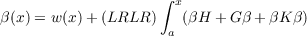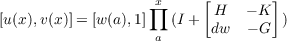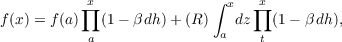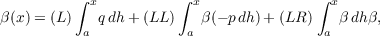#### Vol. 56, No. 1, 1975

 Download this articleFor screen For printingRecent Issues Vol. 325: 1 Vol. 324: 1  2 Vol. 323: 1  2 Vol. 322: 1  2 Vol. 321: 1  2 Vol. 320: 1  2 Vol. 319: 1  2 Vol. 318: 1  2Online Archive Volume: Issue:The Journal Subscriptions Editorial Board Officers Contacts Submission Guidelines Submission Form Policies for Authors ISSN: 1945-5844 (e-only) ISSN: 0030-8730 (print) Special Issues Author Index To Appear Other MSP Journals
A product integral solution of a Riccati equation

### Burrell Washington Helton

Vol. 56 (1975), No. 1, 113–130
##### Abstract

Product integrals are used to show that, if dw, G, H and K are functions from number pairs to a normed complete ring N which are integrable and have bounded variation on [a,b] and v1 exists and is bounded on [a,b], then the integral equationhas a solution β(x) = v1(x)u(x) on [a,b], where u and v are defined by the matrix equationThe above results are used to show that if p,q,h and r are quasicontinuous functions from the numbers to N such that h is left continuous and has bounded variation and p,q and h commute, then the solution on [a,b] of the differential-type equation f∗∗ + fp + fq = r iswhere f(x) f(a) = (L) axfdh, β is the solution ofand z is defined in terms of p,q,r,h and β.

##### Mathematical Subject Classification 2000
Primary: 34A99
Secondary: 26A39, 45A05
##### Milestones